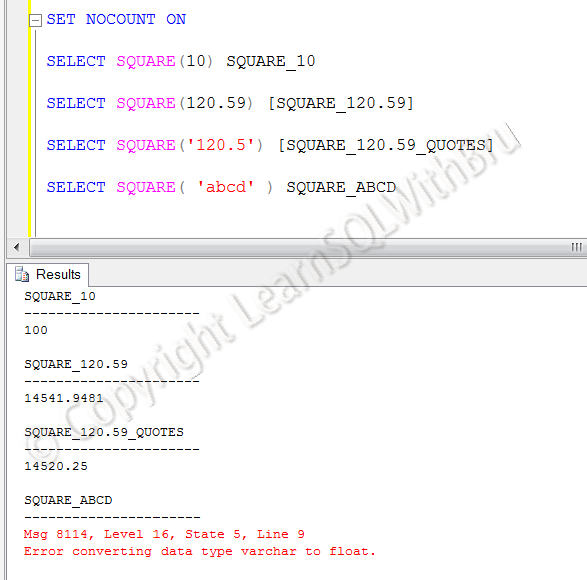# Square – Mathematical function in SQL Server

In this blog post we shall learn a mathematical function in SQL Server called Square. This function expects 1 value to be passed and returns the multiplied value itself nothing but the square of the passed value.

The function expects a value of float data type to be passed. Any other data types, such as an integer will be implicitly converted into a float value. See that the function treats 10 (integer) and 120.59 (float) as float and returns the expected square values..

If the passed value is a integer or a float value with quotes, then that is also converted into a float. See that the function converts ‘120.5’ to float 120.5 without issues.

When the value passed is a datetime or string characters (a – z) or special characters, the could not be converted to float, the function returns an error. See the error below, where ‘abcd’ is passed to the function.Do you like this site? Like our FB page @ Facebook.com\LearnSQLWithBru so that, you know when there is a new blog post.

— Bru Medishetty